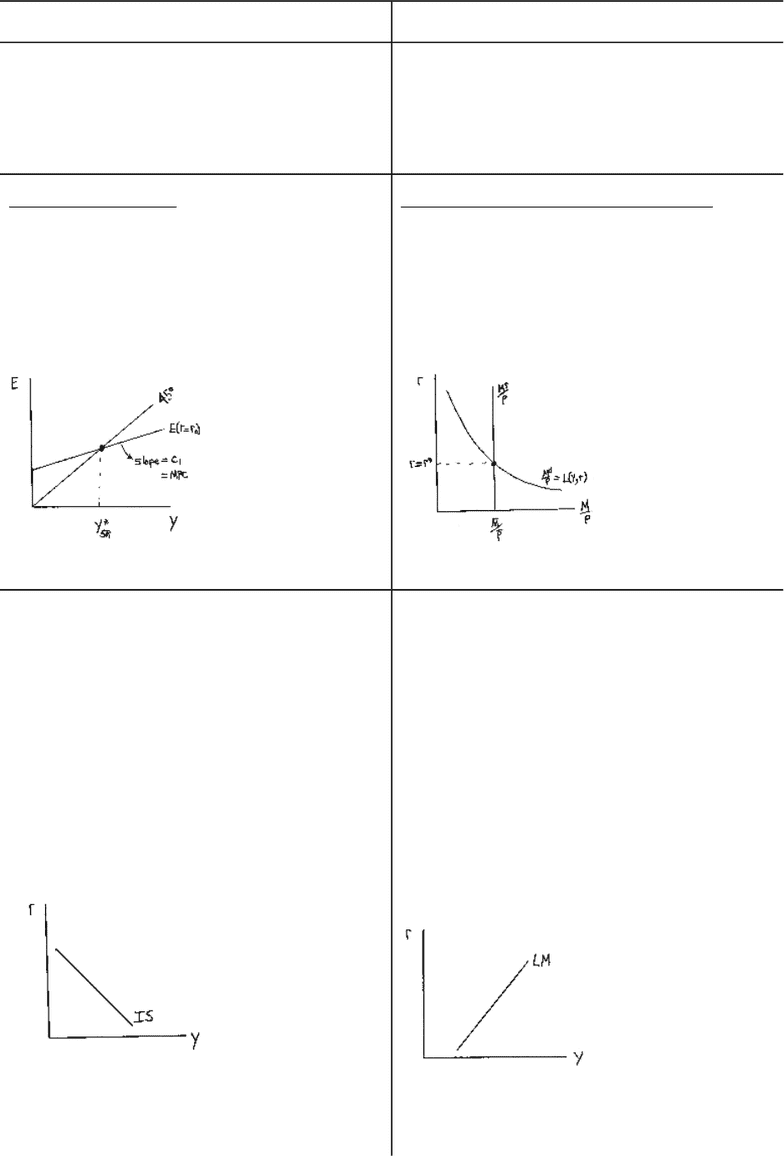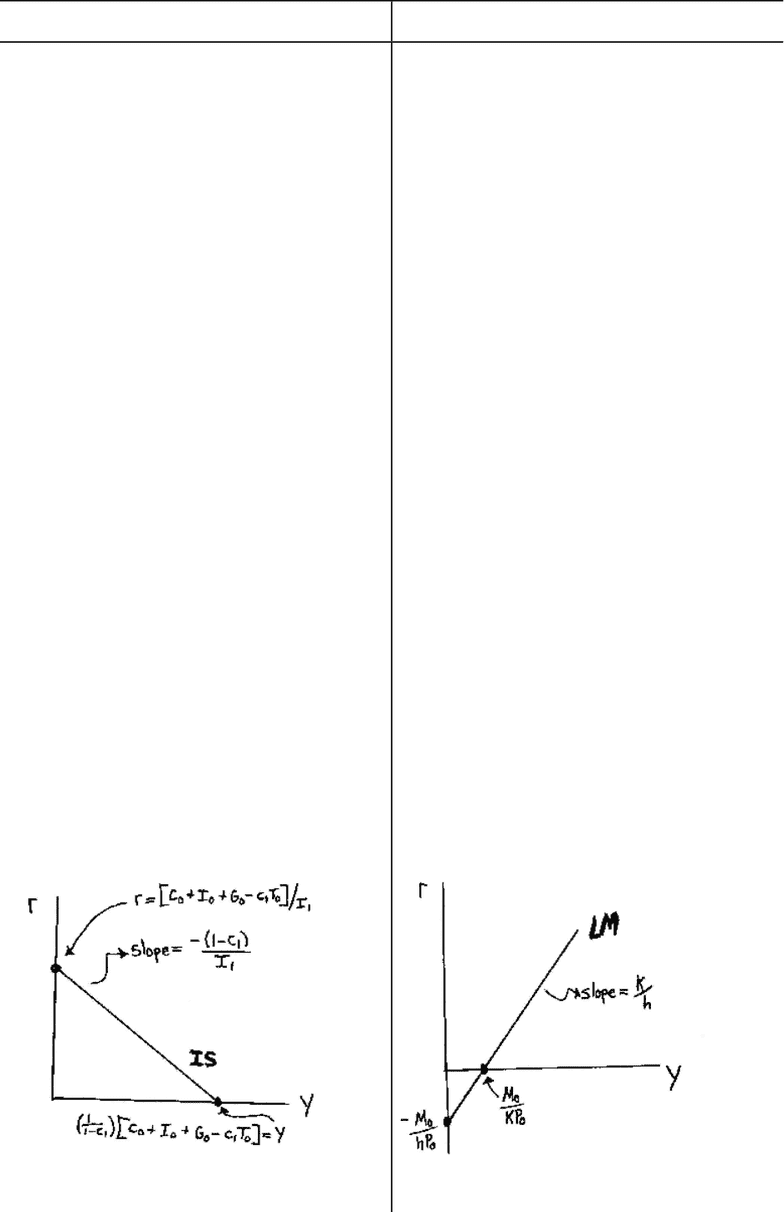# MGEB06H3 Lecture Notes - Lecture 1: Ope, Keynesian Cross, M-Theory

8 views7 pages
School
Course
ProfessorReview of IS/LM Model (Chapters 10 & 11)
IS Curve LM Curve
Ceteris paribus, the Investment Saving or IS
Curve shows all of the (Y,r) pairs that
support (lead to) equilibrium in the Goods and
Services market.
( i.e. YS = YD S = I )
Ceteris paribus, the Liquidity Market or LM
Curve shows all of the (Y,r) pairs that
support (lead to) equilibrium in the Money
(Asset) market.
( i.e. MS = MD AssetD = AssetS )
Keynesian Cross
Short-Run (SR) Equilibrium output (Y*SR)
is determined by equating planned
expenditure (E = YD) to actual expenditure
(Y = YSSR) GIVEN among other things fiscal
policy (i.e. G & T) and the real interest rate
(thus I is fixed).
Theory of Liquidity Preference
The real interest rate varies to determine
equilibrium in the money/assets market
GIVEN output (Y); the money supply (MS);
the aggregate or average price level (P); and
the real money demand curve L(Y,r).
In essence, the IS Curve combines the
interaction between r and I expressed by the
investment function and the interaction
between I and Y demonstrated by the
Keynesian cross.
Having r rise (fall) shifts E down (up) thereby
decreasing (increasing) Y*SR.. This traces out
the IS Curve as downward sloping.
Money market equilibrium implies all asset
markets are in equilibrium. Walras’ Law
states that if all but one market is in
equilibrium then the last market is also.
Therefore, consider assets as consisting of
two types monetary (money) and non-
monetary assets then MS = MD AssetD =
AssetS.
Having Y rise (fall) shifts the real money
demand function ( L(Y,r) ) up (down) thereby
increasing (decreasing) r*SR. This traces out
the LM Curve as upward sloping.
1
Unlock document

This preview shows pages 1-2 of the document.
Unlock all 7 pages and 3 million more documents.IS Curve LM Curve
Algebraic Solution
NX = 0 Closed economy
C = C0 + C1( Y - T ) Consumption Function
I = I0 - I1r Investment Function
G = G0 Exogenous FP
(spending policy)
T = T0Exogenous FP (tax
policy)
Y = C + I + G NII
IS Curve
Y = [ 1/(1-C1) ][ C0 + I0 + G0 - C1T0 ]
- ( I1/(1-C1) )r
derived/drawn for: C0, I0, G0, T0, C1 & I1
given, fixed or exogenous
Y-axis intercept:
Y = [ 1/(1-C1) ][ C0 + I0 + G0 - C1T0 ]
r-axis intercept:
r = [ C0 + I0 + G0 - C1T0 ]/I1
IS Slope: δr/δY = - (1-C1)/I1 < 0
Algebraic Solution
Md = P( kY - hr ) Nom money
demand
Md/P = kY - hr Real money
demand
MS = M0Exogenous MP (exog.
nominal money supply)
P = P0Exogenous or fixed P level
MS = Md or MS/P = Md/P
LM Curve
Y = (1/k)(M0/P0) + ( h/k )r
derived/drawn for: M0S, P0, k & h given,
fixed or exogenous
Y-axis intercept:
Y = (1/k)(M0/P0)
r-axis intercept:
r = ( -1/h )(M0/P0)
LM Slope: δr/δY = k/h > 0
2
Unlock document

This preview shows pages 1-2 of the document.
Unlock all 7 pages and 3 million more documents.

# Get access

\$10 USD/m
Billed \$120 USD annually
Homework Help
Class Notes
Textbook Notes